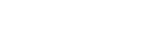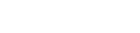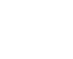# A Geometric and Visual Approach to GMAT Math

Sometimes, the best choice from among two options is neither of them!

## Approaches to the GMAT Quantitative Section

Many quantitative problems contain numbers and demand numerical calculations.  Others contain variables, and one can use either an algebraic approach or a numeric approach.  See this blog post  for more on that choice.  Folks are often aware of these two basic approaches, algebraic and numeric, for attacking GMAT Quant problems.  What folks often forget is that there’s third approach, at least as powerful, which magically unlocks otherwise intractable problems.  First, a sample difficult DS question.

## Sample Problem

1) A, B, P, and Q are four positive numbers.  Does AQ = BP?

Statement #2: In the x-y plane, the line through (A, B) and (P, Q) goes through the origin.

You may want to wrestle with this a bit before reading on.

## I See!

Of course, that third perspective is the geometric/visual perspective.  A gigantic chunk of our cerebral cortex — basically the entire occipital lobe — is devoted to processing visual information.  We homo sapiens are very essentially visual creatures.  Yet, in the mad rush of GMAT Quant, we forget this powerful capacity of ours.  Part of the problem is that Geometry often gets short shrift, both in school and in GMAT review, so we underappreciate what humble branch of math offers.  Algebra we have to calculate.  Geometry we often see immediately.

Of course, in any problem with a diagram, or in any problem that mentioned a geometric shape, you probably will use visual/geometric thinking.  This problem is subtle: only statement #2 even mentions the x-y plane, i.e. coordinate geometry.  As a general rule, when the problem gives even the slightest glimmer that there might be something visual/geometric about it, that is a strong clue that the visual/geometric will yield fast insights.  We will use a visual/geometric approach in solving the above problem.

## Sample Problem Solution

Big geometry idea #1: every point in the x-y plane is the vertex of a unique little right triangle with legs parallel to the axes, with a second vertex on the x-axis and the third vertex at the origin.  The length of the vertical leg is the y-coordinate, and the length of the horizontal leg is the x-coordinate.That one fact has several enormous implications.  Notice, the distance from the point (x,y) to the origin is the hypotenuse, so we could find that distance with the Pythagorean Theorem.This should look familiar.  That’s exactly the form we have for points (A, B) and (P, Q) in statement #1.  The statement:is entirely equivalent to the statement that (A, B) and (P, Q) are equal distances from the origin.  In other words, if we made a circle with center at the origin, we know both points would lie on the same circle.Well, how does this help us answer the question:  Does AQ = BP?  At least in the diagram as it is currently drawn, A and Q are the “big” legs and P and B are the “small” legs, and (big)*(big) ≠ (small)*(small).  For this example, clearly AQ ≠ BP — a “no” answer to the prompt.  But, if A = P and B = Q, the two points would be at the same place.  Statement #1 would still be satisfied, and the answer to the prompt would then be “yes.”  Statement #1 is consistent with both a “yes” answer and “no” answer to the prompt, so by itself it is insufficient.

Now, forget about Statement #1 and focus exclusively on Statement #2.  We are told the line through (A, B) and (P, Q) goes through the origin, like this:Big geometry idea #2: Two triangles of the same shape and same angles but different sizes are called similarSimilar triangles have proportional sides!!  That is one of the deepest and most powerful ideas in all of geometry, and it has staggering implications for problem-solving on the GMAT.

Because the two right triangles are obvious similar, we can set up a proportion among the four sides of interest:Now, cross-multiply:

AQ = PB

Voila!  It turns out: the prompt question is a proportionality question in disguise.  (will you remember that trick on the GMAT?)  Statement #2 allows us to answer the prompt with a definitive “Yes!”, so it is sufficient.  Answer = B.

## Epilogue

The poet Edna St. Vincent Millay (1892 – 1950) wrote: “Euclid alone has looked on beauty bare,” her tribute to the inherent aesthetic appeal of geometry, especially as formulated by its founder, Euclid (c. 300 bce).  Some would argue that the cogent elegance that geometry brings to problem-solving is part and parcel of its aesthetic appeal.  Whatever aesthetic satisfaction you find in geometry, its profound power as a problem-solving tool is undeniable.  Master this perspective, especially the ideas related to similar triangles, and a whole new dimension of problem-solving possibilities will open to you.

## Author

•Mike served as a GMAT Expert at Magoosh, helping create hundreds of lesson videos and practice questions to help guide GMAT students to success. He was also featured as "member of the month" for over two years at GMAT Club. Mike holds an A.B. in Physics (graduating magna cum laude) and an M.T.S. in Religions of the World, both from Harvard. Beyond standardized testing, Mike has over 20 years of both private and public high school teaching experience specializing in math and physics. In his free time, Mike likes smashing foosballs into orbit, and despite having no obvious cranial deficiency, he insists on rooting for the NY Mets. Learn more about the GMAT through Mike's Youtube video explanations and resources like What is a Good GMAT Score? and the GMAT Diagnostic Test.